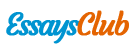# Analysis for the Average Grade of Students at National Economics University in Vietnam

Autor:   •  October 12, 2017  •  2,645 Words (11 Pages)  •  703 Views

Page 1 of 11

...

The expected sign of two remaining variables, the number of absent days and online hours, should be negative. Obviously, missing a class is learning without lecturer and consuming more time for self-study with lower efficient. However, many students will choose the option of skipping this content or waiting until the exam to read again. It results to difficulty in doing task of missed lecture; hence, these students are easy to get a lower mark. The student usually surfs the Internet for entertainment services such as online games, Facebook and YouTube. These activities can help reduce stress, refresh the mind and create better mood for learning. However, these activities usually create addiction for the user. Because, it is so attractive and infinite, the using time often lasts long than the prediction of users and it impact negatively to the average grade. Scientists said that, for students, surfing the Internet has good side and bad side but the negative impact is much more than the positive one.

-

COLLECTION OF DATA

The primary data includes surveys and interviews through a questionnaire. First of all, we create a questionnaire including online and offline version to get the information from a specific students of a group of National Economics University. Then based on student profile developed on the bases of information and data collected, we continue to conduct our project by concretizing and finding out the final results. The specific number of participants is 50 students. The secondary data is obtained through faithful and trustworthy sources of data on the Internet to make the research more informative.

-

REGRESSION RESULTS

The first task is to estimate the population regression function on the basis of the sample regression function as accurately possible. The method of ordinary least square (OLS) is used because it is intuitively appealing and mathematically much simpler than the method of maximum likelihood. Applying OLS method requires some main assumptions. The first one is only applying to the linear model in parameters. Secondly, homoscedasticity or equal variance of ui, the conditional variances of ui are identical. Next, there is no autocorrelation between the disturbances. It is easy to see that the model 1 satisfy the first condition however two other conditions have to be checked to make sure the test for coefficients is reliable.

F-statistic

2.212097

Probability

0.030127

Obs*R-squared

22.20389

Probability

0.052282

Table 1. White Heteroskedasticity Test (cross terms)

Test for heteroscedasticity by using White’s test with cross terms. The null hypothesis is no heteroscedasticity. After estimate model 1 by given data, an auxiliary regression is run:

u^i2 = α1 + α2 SLi + α3 Ai + α4 ONLi + α5 ORIi + α6 SL2i + α7A2i + α8ONL2i + α9SLi*Ai + α10SLi*ONLi + α11SLi*ORIi + α12Ai*ONLi + α13Ai*ORIi + α14ONLi*ORIi + vi

Obtain the R2 from this auxiliary regression and it can be shown that sample size (n) times the R2 obtained from the auxiliary regression asymptotically follows the chi-square distribution with degree of freedom equal to the number of regressors in the auxiliary regression . At 5% level of significance, probability(n.R2), 0.052282, is more than; hence, there is no heteroscedasticity in the estimated regression model of model 1.

The problem of autocorrelation should be concerned. The term autocorrelation may be defined as “correlation between members of series of observations ordered in time [as in time series data] or space [as in cross-sectional data].” However, the phenomenon of autocorrelation usually happens in the time series data. Taking this topic as an example, if cross-sectional data involving the regression of average grade on the amount of self-study hour, the effect of an increase of one student’s self-study hour on his grade is not expected to affect the average grade of another student. Thus, it is possible to assume that the model of average grade has no autocorrelation.

F-statistic

0.375898

Probability

0.688906

Log likelihood ratio

0.866628

Probability

0.648357

Table 2. Ramsey RESET Test

The final problem has to be checked before running estimated model is omitted variables or wrong functional form. Ramsey’s RESET test is conducted to test this problem. From the chosen model, obtain the estimated AGi that is AG^i. Suggesting AG^i2 and AG^i3 as additional regressors; hence, running the auxiliary regression:

AGi = β1 + β2SLi + β3Ai + β4ONLi + β5ORIi + β6 AG^i2 + β7AG^i3 + ui

F-test is conducted for the purpose of dropping two variables AG^i2 and AG^i3. From the result, probability(F-statistic) equals to 0.688906 is more than 5% level of significance; thus, the estimated model of model 1 is not mis-specified.

Dependent Variable: AG

Method: Least Squares

Sample: 1 50

Included observations: 50

Variable

Coefficient

Std. Error

t-Statistic

Prob.

C

8.270150

0.197787

41.81351

0.0000

SL

0.071240

0.029469

2.417482

0.0197

A

...